### IMO Shortlist 2008 problem N5

Kvaliteta:
Avg: 0,0
Težina:
Avg: 8,0
For every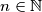$n\in\mathbb{N}$ let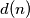$d(n)$ denote the number of (positive) divisors of$n$. Find all functions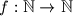$f: \mathbb{N}\to\mathbb{N}$ with the following properties: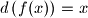$d\left(f(x)\right) = x$ for all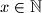$x\in\mathbb{N}$.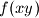$f(xy)$ divides$(x - 1)y^{xy - 1}f(x)$ for all$x$,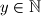$y\in\mathbb{N}$.

Proposed by Bruno Le Floch, France
Izvor: Međunarodna matematička olimpijada, shortlist 2008